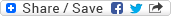## On some classes of q-plurisubharmonic functions and q-pseudoconcave sets

### Dateibereich 5186

1,18 MB in einer Datei, zuletzt geändert am 22.01.2018

DateiDateien geändert amGröße
dc1526.pdf22.01.2018 13:11:371,18 MB

We introduce real q-convex functions on open subsets of the real Euclidean space. We study their properties and develop approximation techniques by continuous ones and by those who are real q-convex with corners. In the smooth case, a function is real q-convex if and only if its real Hessian has at most q negative eigenvalues. We compare these functions to their complex relatives: the q-plurisubharmonic functions defined on open sets of the complex Euclidean space. We show that each real q-convex function is q-plurisubharmonic. On the converse, each q-plurisubharmonic function, which is invariant in the imaginary part, is real q-convex. Another example for q-plurisubharmonic functions is given by those which are plurisubharmonic on leaves of a singular foliation by analytic sets of codimension q.

The q-plurisubharmonic functions are used to define q-pseudoconcave sets. These are closed subsets S of an open set D in the complex Euclidean space for which the negative logarithm of the distance function to S is q-plurisubharmonic on D minus S. We prove that (Hartogs) q-pseudoconcave graphs of certain continuous mappings locally admit a foliation by complex submanifolds of dimension q. We construct generalized convex hulls of compact sets with respect to subfamilies of q-plurisubharmonic functions and compare them to each other and to hulls already existing in the literature (such as Basener's hull or the rationally convex hull).

We verify that the Shilov boundary of a compact set for certain families of upper semi-continuous functions exists and coincides with the set of all peak points for the given family. As an application, we study the Shilov boundary of a convex body for q-plurisubharmonic functions and generalize Bychkov's result from 1981. More precisely, the complement of the Shilov q-boundary of a convex body K consists of all boundary points p with the following property: there exists an open neighbourhood U of p in the boundary of K such that each point z in U lies in some open part of a complex plane of dimension at least q+1.

Lesezeichen:
Permalink |Dokumententyp:
Wissenschaftliche Abschlussarbeiten » Dissertation
Fakultäten und Einrichtungen:
Fakultät für Mathematik und Naturwissenschaften » Mathematik und Informatik » Dissertationen
Dewey Dezimal-Klassifikation:
500 Naturwissenschaften und Mathematik » 510 Mathematik
Sprache:
Englisch
Kollektion / Status:
Dissertationen / Dokument veröffentlicht
Dateien geändert am:
22.01.2018
Datum der Promotion:
24.11.2015
Medientyp:
Text